International
Tables for
Crystallography
Volume A
Space-group symmetry
Edited by M. I. Aroyo

International Tables for Crystallography (2016). Vol. A, ch. 1.7, pp. 136-139

Section 1.7.3. Relationships between space groups and subperiodic groups

D. B. Litvinc and V. Kopskýd

1.7.3. Relationships between space groups and subperiodic groups

| top | pdf |

The present volume in the series International Tables for Crystallography (Volume A: Space-Group Symmetry) treats one-, two- and three-dimensional space groups. Volume E in the series, Subperiodic Groups (2010), treats two- and three-dimensional subperiodic groups: frieze groups (groups in two-dimensional space with translations in a one-dimensional subspace), rod groups (groups in three-dimensional space with translations in a one-dimensional subspace) and layer groups (groups in three-dimensional space with translations in a two-dimensional subspace). In the same way in which three-dimensional space groups are used to classify the atomic structure of three-dimensional crystals, the subperiodic groups are used to classify the atomic structure of other crystalline structures, such as liquid crystals, domain interfaces, twins and thin films.

In Volume A, the relationship between the space group of a crystal and the point-group symmetry of individual points in the crystal is given by site symmetries, the point-group subgroups of the space group that leave the points invariant. In Volume E, an analogous relationship is given between the space group of a crystal and the subperiodic-group symmetry of planes that transect the crystal. Volume E contains scanning tables (with supplementary tables in Kopský & Litvin, 2004) from which the layer-group subgroups of the space group (called sectional layer groups) that leave the transecting planes invariant can be determined. The first attempts to derive sectional layer groups were made by Wondratschek (1971) and by using software written by Guigas (1971). Davies & Dirl (1993a,b) developed software for finding subgroups of space groups which was modified to find sectional layer groups. The use and determination of sectional layer groups have also been discussed by Janovec et al. (1988), Kopský & Litvin (1989) and Fuksa et al. (1993).

In Fig. 1.7.3.1, part of the scanning table for the space group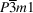(164) is given. From this one can determine the layer-group subgroups ofthat are symmetries of planes of orientation (hkil) = (0001). Vectors a′ and b′ are basic vectors of the translational subgroup of the layer-group symmetry of planes of this orientation. The vector d defines the scanning direction and is used to define the position of the plane within the crystal. The linear orbit is the set of all parallel planes obtained by applying all elements of the space group to any one plane. The sectional layer group is the layer subgroup of the space group that leaves the plane invariant.Figure 1.7.3.1 | top | pdf |The scanning table for the space-group type(164) and orientation orbit (0001), and the structure of cadmium iodide, CdI2. Cadmium and iodine ions are denoted by open and filled circles, respectively.

Sectional layer groups were introduced by Holser (1958a,b) in connection with the consideration of domain walls and twin boundaries as symmetry groups of planes bisecting a crystal. The mutual orientation of the two domains separated by a domain wall or twin boundary is not arbitrary, but has crystallographic restrictions. The group-theoretical basis for an analysis of domain pairs is given by Janovec (1972), and the structure of domain walls and twin boundaries is considered by Janovec (1981) and Zikmund (1984) [see also Janovec & Přívratská (2014)].

Layer symmetries have been used in bicrystallography. The term bicrystal was introduced by Pond & Bollmann (1979) in the study of grain boundaries [see also Pond & Vlachavas (1983) and Vlachavas (1985)]. A bicrystal is in general an edifice where two crystals, usually of the same structure but of different, possibly arbitrary, orientations, meet at a common boundary. The sectional layer groups describe the symmetries of such a boundary [see Volume E (2010), Section 5.2.5.2].

An example of the application of the scanning tables to determine the layer-group symmetry of planes in a crystal is given in Section 1.7.3.1. In Section 1.7.3.2the derivation of the layer-group symmetry of a domain wall is described.

1.7.3.1. Layer symmetries in three-dimensional crystal structures

| top | pdf |

Fig. 1.7.3.1shows the crystal structure of cadmium iodide, CdI2. The space group of this crystal is of type(164). The anions form a hexagonal close packing of spheres and the cations occupy half of the octahedral holes, filling one of the alternate layers. In close-packing notation, the CdI2 structure is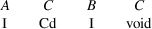From the scanning tables, we obtain for planes with the (0001) orientation and at heights z = 0 or z = ½ a sectional layer-group symmetry type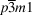(layer group No. 72, or L72 for short), and for planes of this orientation at any other height a sectional layer-group symmetry type p3m1 (L69).

The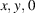plane contains cadmium ions. This plane is a constituent of the orbit of planes of orientation (0001) passing through the points with coordinates 0, 0, u, where u is an integer. All these planes contain cadmium ions in the same arrangement (C layer filled with Cd).

The plane at height z = ½ is a constituent of the orbit of planes of orientation (0001) passing through the points with coordinates 0, 0, u + ½. All these planes contain only voids and lie midway between A and B layers of iodine ions with the B layer below and the A layer above the plane.

The planes at levels z = ¼ and z = ¾ contain B and A layers of iodine ions, respectively. These planes and all planes related to them by translations t(0, 0, u) belong to the same orbit because the operations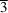exchange the A and B layers.

1.7.3.2. The symmetry of domain walls

| top | pdf |

The cubic phase of barium titanate BaTiO3, of symmetry type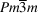, undergoes a phase transition to a tetragonal phase of symmetry typewhich can give rise to six distinct single-domain states (Janovec et al., 2004). This is represented in Fig. 1.7.3.2, where at the centre are four unit cells of the cubic phase with barium and titanium atoms represented by large and small filled circles, respectively, and oxygen atoms, which are located at the centre of each unit-cell face, as open circles. A cubic-to-tetragonal phase transition gives rise to atomic displacements represented by arrows, and to six single-domain states, four of which are depicted in the figure. The polar tetragonal symmetry of each of these tetragonal domain states is also shown.Figure 1.7.3.2 | top | pdf |At the centre is the structure of the cubic phase of barium titanate, BaTiO3, of symmetry type, surrounded by the structures of four of the six single-domain states of the tetragonal phase of symmetry type P4mm. All the diagrams are projections along the  direction. Arrows depict the atomic displacement amplitudes from their cubic-phase positions.

In determining the symmetry of a domain wall, we first construct a domain twin (Janovec & Přívratská, 2014): we choose two single-domain states, for this example the two in Fig. 1.7.3.2with symmetry P4zmxmxy, and construct a domain pair consisting of the superposition of these two single-domain states, see Fig. 1.7.3.3. The domain twin we choose to construct is obtained by passing a plane of orientation (010) through this domain pair at the origin and deleting from one side of the plane the atoms of one of the single-domain states, and the atoms of the second single-domain state from the other side of the plane, see Fig. 1.7.3.4. The plane is referred to as the central plane of the domain wall, and the atoms in and near this plane as the domain wall.Figure 1.7.3.3 | top | pdf |The domain pair of symmetry P4z/mzmxmxy consisting of the superposition of those two single-domain states of tetragonal symmetry P4zmxmxy shown in Fig. 1.7.3.2. The diagram is a projection along the  direction.

The symmetry of the central plane of the domain wall is determined from the symmetry of the domain pair and the scanning tables: The symmetry of the domain pair is the group of operations that either leaves both single-domain states invariant or simultaneously switches the two domain states. P4zmxmxy leaves both single-domain states invariant and the symmetry operation of spatial inversion switches the two single-domain states, see Fig. 1.7.3.3. Consequently, the domain-pair symmetry is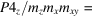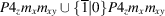. The symmetry of the central plane is determined from the scanning table for the space group P4z/mzmxmxy, the orientation orbit (010), the orientation of the domain wall and the linear orbit 0d, since the central plane of the wall passes through the origin (Volume E, 2010). The symmetry of the central plane is the sectional layer group pmxmzmy, where p denotes the lattice of translations in the x, 0, z plane.

Let n denote a unit vector perpendicular to the central plane of the domain wall; in this example n is in the  direction. The symmetry of the domain wall consists of:

 (1) all elements of the symmetry group of the central plane that leave n and both domain states invariant, i.e. in this example, all translations of p, 1 and mx; and (2) all elements of the symmetry group of the central plane that invert n and switch the domain states, i.e. in this example, 2x and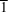.

The symmetry of the domain wall is then p2x/mx.

References

International Tables for Crystallography (2010). Vol. E, Subperiodic Groups, edited by V. Kopský & D. B. Litvin, 2nd ed. Chichester: John Wiley & Sons.
Davies, B. L. & Dirl, R. (1993a). Space-group subgroups generated by sublattice relations: software of IBM-compatible PCs. Anales de Física, Mongrafías, Vol. 2, edited by M. A. del Olmo, M. Santander & J. M. Mateos Guilarte, pp. 338–341. Madrid: CIEMAT/RSEF.
Davies, B. L. & Dirl, R. (1993b). Space-group subgroups, coset decompositions, layer and rod symmetries: integrated software for IBM-compatible PCs. Third Wigner Colloquium, Oxford, September 1993.
Fuksa, J., Kopský, V. & Litvin, D. B. (1993). Spatial distribution of rod and layer symmetries in a crystal. Anales de Física, Mongrafías, Vol. 2, edited by M. A. del Olmo, M. Santander & J. M. Mateos Guilarte, pp. 346–369. Madrid: CIEMAT/RSEF.
Guigas, B. (1971). PROSEC. Institut für Kristallographie, Universtität Karlsruhe, Germany. (Unpublished.)
Holser, W. T. (1958a). The relation of structure to symmetry in twinning. Z. Kristallogr. 110, 249–263.
Holser, W. T. (1958b). Point groups and plane groups in a two-sided plane and their subgroups. Z. Kristallogr. 110, 266–281.
Janovec, V. (1972). Group analysis of domains and domain pairs. Czech. J. Phys. 22, 974–994.
Janovec, V. (1981). Symmetry and structure of domain walls. Ferroelectrics, 35, 105–110.
Janovec, V., Grocký, M., Kopský, V. & Kluiber, Z. (2004). On atomic displacements in 90° ferroelectric domain walls of tetragonal BaTiO3 crystals. Ferroelectrics, 303, 65–68.
Janovec, V., Kopský, V. & Litvin, D. B. (1988). Subperiodic subgroups of space groups. Z. Kristallogr. 185, 282.
Janovec, V. & Přívratská, J. (2014). Domain structures. In International Tables for Crystallography, Vol. D, Physical Properties of Crystals, edited by A. Authier, 2nd ed., ch. 3.4. Chichester: Wiley.
Kopský, V. & Litvin, D. B. (1989). Scanning of space groups. In Group Theoretical Methods in Physics, edited by Y. Saint Aubin & L. Vinet, pp. 263–266. Singapore: World Scientific.
Kopský, V. & Litvin, D. B. (2004). Space-group scanning tables. Acta Cryst. A60, 637.
Locherer, K. R., Swainson, I. P. & Salje, E. K. H. (1999). Transition to a new tetragonal phase of WO3: crystal structure and distortion parameters. J. Phys. Condens. Matter, 11, 4143–4156.
Pond, R. C. & Bollmann, W. (1979). The symmetry and interfacial structure of bicrystals. Philos. Trans. R. Soc. London Ser. A, 292, 449–472.
Pond, R. C. & Vlachavas, D. S. (1983). Bicrystallography. Proc. R. Soc. London Ser. A, 386, 95–143.
Vlachavas, D. S. (1985). Symmetry of bicrystals corresponding to a given misorientation relationship. Acta Cryst. A41, 371–376.
Wondratschek, H. (1971). Institut für Kristallographie, Universtität Karlsruhe, Germany. (Unpublished.)
Zikmund, Z. (1984). Symmetry of domain pairs and domain twins. Czech. J. Phys. 34, 932–949.